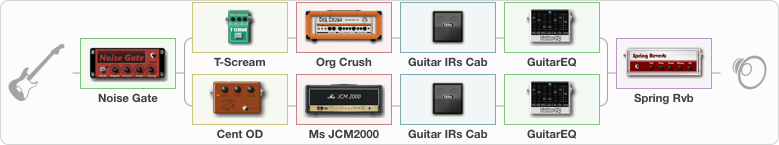# High gain tone

Discussion in 'ToneLib-GFX presets' started by Pablo Pso Studios, Feb 17, 2021.

1. High gain tone

Preset name: High gain tone

Sounds best with active humbuckers

Effects chain:Effect: "Noise Gate" (Dynamics / Filter), active - "yes"
{
"Mode" = Auto
"Depth" = 50
"Threshold" = 32
"Attack" = 27
"Hold" = 5
"Decay" = 58
}

Effect: "Splitter" (Dynamics / Filter), active - "yes"
{
"A-Bypass" = Off
"A-Pan" = 100
"A-Level" = 61
"B-Bypass" = Off
"B-Pan" = -100
"B-Level" = 65

'A' branch:
{

Effect: "T-Scream" (Overdrive / Distortion), active - "yes"
{
"Drive" = 13
"Tone" = 80
"Level" = 79
}

Effect: "Org Crush" (Amp simulators), active - "yes"
{
"Gain" = 46
"Bass" = 42
"Middle" = 62
"Treble" = 38
"Presence" = 61
"Master" = 50
"Level (dB)" = 0
}

Effect: "Guitar IRs Cab" (Cabinets), active - "yes"
{
"Model" = Orange PPC (4x12")
"Mic Position" = Middle
"Mic Distance" = Near
"Low Cut (Hz)" = 60
"Hi Cut (kHz)" = 20.0
"Mix" = 100
"Level (dB)" = 0
}

Effect: "GuitarEQ" (Dynamics / Filter), active - "yes"
{
"160 Hz" = -5
"400 Hz" = -4
"800 Hz" = -2
"1.6 kHz" = 3
"3.2 kHz" = -3
"6.4 kHz" = -2
"12 kHz" = -4
"Level (dB)" = 0
}
}
'B' branch:
{

Effect: "Cent OD" (Overdrive / Distortion), active - "yes"
{
"Drive" = 41
"Tone" = 76
"Level" = 38
}

Effect: "Ms JCM2000" (Amp simulators), active - "yes"
{
"Gain" = 52
"Bass" = 36
"Middle" = 58
"Treble" = 38
"Presence" = 63
"Master" = 50
"Level (dB)" = 0
}

Effect: "Guitar IRs Cab" (Cabinets), active - "yes"
{
"Model" = Marshall JCM2000 (4x12")
"Mic Position" = Middle
"Mic Distance" = Near
"Low Cut (Hz)" = 60
"Hi Cut (kHz)" = 20.0
"Mix" = 100
"Level (dB)" = 0
}

Effect: "GuitarEQ" (Dynamics / Filter), active - "yes"
{
"160 Hz" = -4
"400 Hz" = -3
"800 Hz" = -2
"1.6 kHz" = -2
"3.2 kHz" = 4
"6.4 kHz" = -1
"12 kHz" = -3
"Level (dB)" = 0
}
}
}

Effect: "Spring Rvb" (Reverberation), active - "yes"
{
"Time" = 5.6
"PreDelay" = 9
"LoDamp" = 23
"HiDamp" = 38
"Mix" = 23
}

Note: You will need to download and install the ToneLib-GFX software to use the preset.

File size:
1.1 KB
Views:
2,705